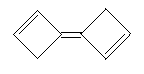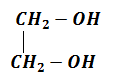$\underset{\mathrm{Zn}/\mathrm{AcOH}}{\overset{{\mathrm{O}}_{3}}{\to }}$A
A is:

1. 3-keto butanol

2. Butan-1,4-dial

3. 1-aldo-2-butanone

4. 2-keto butan-1,4-dial

Concept Questions :-

Alkanes, Alkenes and Alkynes - Chemical Properties
High Yielding Test Series + Question Bank - NEET 2020

Difficulty Level:

A compound 'A'

1. Decolourises Baeyer's reagent

2. On hydroxylation and futher oxidation with ${\mathrm{KMnO}}_{4}$ gave formic acid and acetone

3. On ozonolysis gave one molecule of acetone and one of formaldehyde 'A' is

1. Propylene            2. Ethylene

3. n-butylene           4. Isobutylene

Concept Questions :-

Alkanes, Alkenes and Alkynes - Chemical Properties
High Yielding Test Series + Question Bank - NEET 2020

Difficulty Level:

The most reactive of these compounds towards sulphonation is

1. Toluene                         2. Chlorobenzene

3. Nitrobenzene                 4. m-Xylene

Concept Questions :-

Aromatic Hydrocarbons - Functional Groups, Directive Influence in Monosubstituted Benzene
High Yielding Test Series + Question Bank - NEET 2020

Difficulty Level:

${\mathrm{CH}}_{3}\mathrm{Cl}\stackrel{\mathrm{Na}}{\to }$Gas A

${\mathrm{CH}}_{2}\mathrm{COONa}-{\mathrm{CH}}_{2}\mathrm{COONa}$$\underset{\mathrm{Electrolysis}}{\overset{\mathrm{Kolbe}\text{'}\mathrm{s}}{\to }}$Gas B

${\mathrm{Mg}}_{2}{\mathrm{C}}_{3}\stackrel{{\mathrm{H}}_{2}\mathrm{O}}{\to }$Gas C

Gas (A+B+C) $\underset{{\mathrm{Cu}}_{2}{\mathrm{Cl}}_{2}}{\overset{\mathrm{Ammoniacal}}{\to }}$

gas mixture  Gas X

Gas X is same is

1. Gas A + Gas B                      2. Only Gas B

3. Gas B + Gas C                      4. Only Gas A

Concept Questions :-

Alkanes, Alkenes and Alkynes -Methods of Preparation
High Yielding Test Series + Question Bank - NEET 2020

Difficulty Level:

The product obtained on heating n-heptane with ${\mathrm{Cr}}_{2}{\mathrm{O}}_{3}-{\mathrm{Al}}_{2}{\mathrm{O}}_{3}$ at $600°\mathrm{C}$ is

1. cyclohexane                               2. cyclohexene

3. benzene                                    4. toluene

Concept Questions :-

Alkanes, Alkenes and Alkynes - Nomenclature and Isomerism
High Yielding Test Series + Question Bank - NEET 2020

Difficulty Level:

The intermediate during the addition of HCl to propene in the presence of peroxide is

1. ${\mathrm{CH}}_{3}\stackrel{*}{\mathrm{C}}{\mathrm{HCH}}_{2}\mathrm{Cl}$                      2. ${\mathrm{CH}}_{3}\stackrel{+}{\mathrm{C}}{\mathrm{HCH}}_{3}$

3. ${\mathrm{CH}}_{3}{\mathrm{CH}}_{2}\stackrel{*}{{\mathrm{CH}}_{2}}$                        4. ${\mathrm{CH}}_{3}{\mathrm{CH}}_{2}\stackrel{+}{{\mathrm{CH}}_{2}}$

Concept Questions :-

Alkanes, Alkenes and Alkynes - Chemical Properties
High Yielding Test Series + Question Bank - NEET 2020

Difficulty Level:

Which reagent distinguish propyne and propene?

1. Alkaline ${\mathrm{KMnO}}_{4}$

2. ${\mathrm{Cu}}_{2}{\mathrm{Cl}}_{2}$ + ${\mathrm{NH}}_{4}\mathrm{Cl}$

3. ${\mathrm{Br}}_{2}$ water

4. ${\mathrm{Cu}}_{2}{\mathrm{Cl}}_{2}$ + ${\mathrm{NH}}_{4}\mathrm{OH}$

Concept Questions :-

Alkanes, Alkenes and Alkynes - Chemical Properties
High Yielding Test Series + Question Bank - NEET 2020

Difficulty Level:

When propane is reacted with conc. ${\mathrm{HNO}}_{3}$ at $400-500°\mathrm{C}$ the number of nitro alkanes obtained are

1. 1                              2. 2

3. 3                              4. 4

Concept Questions :-

Alkanes, Alkenes and Alkynes - Nomenclature and Isomerism
High Yielding Test Series + Question Bank - NEET 2020

Difficulty Level:

$\mathrm{CH}\equiv \mathrm{CH}\stackrel{{\mathrm{O}}_{3}/\mathrm{NaOH}}{\to }$X$\stackrel{\mathrm{Zn}/{\mathrm{CH}}_{3}\mathrm{COOH}}{\to }$Y

Y is:

1.2. ${\mathrm{CH}}_{3}{\mathrm{CH}}_{2}\mathrm{OH}$

3. ${\mathrm{CH}}_{3}\mathrm{COOH}$                       4. ${\mathrm{CH}}_{3}\mathrm{OH}$

Concept Questions :-

Alkanes, Alkenes and Alkynes - Chemical Properties
High Yielding Test Series + Question Bank - NEET 2020

Difficulty Level:

Which alkene has smallest heat of hydrogenation?

1. ${\mathrm{CH}}_{3}-\mathrm{CH}=\mathrm{CH}-{\mathrm{CH}}_{3}$

2. ${\left({\mathrm{CH}}_{3}\right)}_{2}\mathrm{C}=\mathrm{CH}-{\mathrm{CH}}_{3}$

3. ${\mathrm{CH}}_{2}=\mathrm{CH}-{\mathrm{CH}}_{2}-{\mathrm{CH}}_{3}$

4. ${\mathrm{CH}}_{3}-\mathrm{CH}=\mathrm{CH}-\mathrm{CH}{\left({\mathrm{CH}}_{3}\right)}_{2}$

Concept Questions :-

Alkanes, Alkenes and Alkynes - Chemical Properties